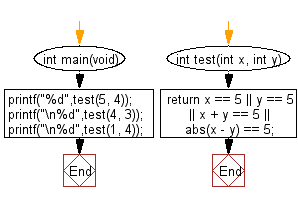﻿ C : 2 integers, if one is 5 or their sum or difference is 5# C Exercises: Accept two integers and return true if either one is 5 or their sum or difference is 5

## C-programming basic algorithm: Exercise-18 with Solution

Write a C program that accept two integers and return true if either one is 5 or their sum or difference is 5.

C Code:

``````#include <stdio.h>
#include <stdlib.h>

int main(void){
printf("%d",test(5, 4));
printf("\n%d",test(4, 3));
printf("\n%d",test(1, 4));
}

int test(int x, int y)
{
return x == 5 || y == 5 || x + y == 5 || abs(x - y) == 5;
}
``````

Sample Output:

```1
0
1
```

Pictorial Presentation:Flowchart:C Programming Code Editor:

What is the difficulty level of this exercise?

Test your Programming skills with w3resource's quiz.

﻿

## C Programming: Tips of the Day

Why doesn't a+++++b work?

printf("%d",a+++++b); is interpreted as (a++)++ + b according to the Maximal Munch Rule!.

++ (postfix) doesn't evaluate to an lvalue but it requires its operand to be an lvalue.

! 6.4/4 says the next preprocessing token is the longest sequence of characters that could constitute a preprocessing token"

Ref : https://bit.ly/3fdldUT